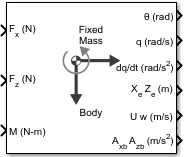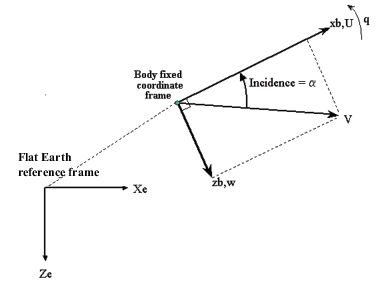# 3DOF (Body Axes)

Implement three-degrees-of-freedom equations of motion with respect to body axes

• Library:
• Aerospace Blockset / Equations of Motion / 3DOF

•## Description

The 3DOF (Body Axes) block implements three-degrees-of-freedom equations of motion with respect to body axes. It considers the rotation in the vertical plane of a body-fixed coordinate frame about a flat Earth reference frame. For more information about the rotation and equations of motion, see Algorithms.

## Ports

### Input

expand all

Applied force along the body x-axis, specified as a scalar, in the units selected in Units.

Data Types: `double`

Applied force along the body z-axis, specified as a scalar.

Data Types: `double`

Applied pitching moment, specified as a scalar.

Data Types: `double`

Gravity, specified as a scalar.

#### Dependencies

To enable this port, set Gravity source to `External`.

Data Types: `double`

### Output

expand all

Pitch attitude, within ±pi, returned as a scalar, in radians.

Data Types: `double`

Pitch angular rate, returned as a scalar, in radians per second.

Data Types: `double`

Pitch angular acceleration, returned as a scalar, in radians per second squared.

Data Types: `double`

Location of the body in the flat Earth reference frame, (Xe, Ze), returned as a two-element vector.

Data Types: `double`

Velocity of the body resolved into the body-fixed coordinate frame, (u, w), returned as a two-element vector.

Data Types: `double`

Acceleration of the body with respect to the body-fixed coordinate frame, (Ax, Az), returned as a two-element vector.

Data Types: `double`

Accelerations of the body with respect to the inertial (flat Earth) coordinate frame, returned as a two-element vector. You typically connect this signal to the accelerometer.

#### Dependencies

To enable this port, select the Include inertial acceleration check box.

Data Types: `double`

## Parameters

expand all

### Main

Input and output units, specified as `Metric (MKS)`, `English (Velocity in ft/s)`, or `English (Velocity in kts)`.

UnitsForcesMomentAccelerationVelocityPositionMassInertia
`Metric (MKS)` NewtonNewton-meterMeters per second squaredMeters per secondMetersKilogramKilogram meter squared
`English (Velocity in ft/s)` PoundFoot-poundFeet per second squaredFeet per secondFeetSlugSlug foot squared
`English (Velocity in kts)` PoundFoot-poundFeet per second squaredKnotsFeetSlugSlug foot squared

#### Programmatic Use

 Block Parameter: `units` Type: character vector Values: `Metric (MKS)` | `English (Velocity in ft/s)` | `English (Velocity in kts)` Default: `Metric (MKS)`

Body or wind axes, specified as `Wind` or `Body`

#### Programmatic Use

 Block Parameter: `axes` Type: character vector Values: `Wind` | `Body` Default: `Body`

Mass type, specified according to the following table.

Mass TypeDescriptionDefault for
`Fixed`

Mass is constant throughout the simulation.

`Simple Variable`

Mass and inertia vary linearly as a function of mass rate.

`Custom Variable`

Mass and inertia variations are customizable.

The `Fixed` selection conforms to the previously described equations of motion.

#### Programmatic Use

 Block Parameter: `mtype` Type: character vector Values: `Fixed` | `Simple Variable` | `Custom Variable` Default: `'Fixed'`

Initial velocity of the body, (V0), specified as a scalar.

#### Programmatic Use

 Block Parameter: `v_ini` Type: character vector Values: `'100'` | scalar Default: `'100'`

Initial pitch attitude of the body, (θ0), specified as a scalar.

#### Programmatic Use

 Block Parameter: `theta_ini` Type: character vector Values: `'0'` | scalar Default: `'0'`

Initial pitch rotation rate, (q0), specified as a scalar.

#### Programmatic Use

 Block Parameter: `q_ini` Type: character vector Values: `'0'` | scalar Default: `'0'`

Initial angle between the velocity vector and the body, (α0), specified as a scalar.

#### Programmatic Use

 Block Parameter: `alpha_ini` Type: character vector Values: `'0'` | scalar Default: `'0'`

Initial location of the body in the flat Earth reference frame, specified as a two-element vector.

#### Programmatic Use

 Block Parameter: `pos_ini` Type: character vector Values: `'[0 0]'` | two-element vector Default: `'[0 0]'`

Initial mass of the rigid body, specified as a scalar.

#### Programmatic Use

 Block Parameter: `mass` Type: character vector Values: `'1.0'` | scalar Default: `'1.0'`

Inertia of the body, specified as a scalar.

#### Dependencies

To enable this parameter, set Mass type to `Fixed`.

#### Programmatic Use

 Block Parameter: `Iyy` Type: character vector Values: `'1.0'` | scalar Default: `'1.0'`

Gravity source, specified as:

 `External` Variable gravity input to block `Internal` Constant gravity specified in mask

#### Programmatic Use

 Block Parameter: `g_in` Type: character vector Values: `'Internal'` | `'External'` Default: `'Internal'`

Acceleration due to gravity, specified as a double scalar and used if internal gravity source is selected. If gravity is to be neglected in the simulation, this value can be set to `0`.

#### Dependencies

• To enable this parameter, set Gravity Source to `Internal`.

#### Programmatic Use

 Block Parameter: `g` Type: character vector Values: `'9.81'` | scalar Default: `'9.81'`

Select this check box to add an inertial acceleration in flat Earth frame output port. You typically connect this signal to the accelerometer.

#### Dependencies

To enable the AxeAze port, select this parameter.

#### Programmatic Use

 Block Parameter: `abi_flag` Type: character vector Values: `'off'` | `'on'` Default: `'off'`

### State Attributes

Assign a unique name to each state. You can use state names instead of block paths during linearization.

• The number of names must match the number of states, as shown for each item, or be empty. Set all or none of the block states.

• To assign names to single-variable states, enter unique names between quotes, for example, `'q'` or `"q"`.

• To assign names to two-variable states, enter a comma-separated list surrounded by braces, for example, `{'Xe','Ze'}`.

• If a state parameter is empty (`' '`), no name is assigned.

• To assign state names with a variable in the MATLAB® workspace, enter the variable without quotes. A variable can be a character vector, cell array of character vectors, or string.

Velocity state names, specified as a comma-separated list surrounded by braces.

#### Programmatic Use

 Block Parameter: `vel_statename` Type: character vector Values: `''` | comma-separated list surrounded by braces Default: `''`

Position state names, specified as a comma-separated list surrounded by braces.

#### Programmatic Use

 Block Parameter: `pos_statename` Type: character vector Values: `''` | comma-separated list surrounded by braces Default: `''`

Pitch angular rate state name, specified as a character vector or string.

#### Programmatic Use

 Block Parameter: `q_statename` Type: character vector | string Values: `''` | scalar Default: `''`

Pitch attitude state name, specified as a character vector or string.

#### Programmatic Use

 Block Parameter: `theta_statename` Type: character vector | string Values: `''` Default: `''`

## Algorithms

The block considers the rotation in the vertical plane of a body-fixed coordinate frame about a flat Earth reference frame.The equations of motion are

`$\begin{array}{l}{A}_{xb}=\stackrel{˙}{u}={A}_{xe}-qw\\ {A}_{zb}=\stackrel{˙}{w}={A}_{ze}+qu\\ {A}_{xe}=\frac{{F}_{x}}{m}-g\mathrm{sin}\theta \\ {A}_{ze}=\frac{{F}_{z}}{m}+g\mathrm{cos}\theta \\ {\stackrel{˙}{X}}_{e}=u\mathrm{cos}\theta +w\mathrm{sin}\theta \\ {\stackrel{˙}{Z}}_{e}=-u\mathrm{sin}\theta +w\mathrm{cos}\theta \\ \stackrel{˙}{q}=\frac{{M}_{y}}{{I}_{yy}}\\ \stackrel{˙}{\theta }=q\end{array}$`

where the applied forces are assumed to act at the center of gravity of the body. Input variables are Fx, Fz, My. g is an optional input variable.

## Compatibility Considerations

expand all

Behavior changed in R2021b

## Extended Capabilities

### C/C++ Code GenerationGenerate C and C++ code using Simulink® Coder™.